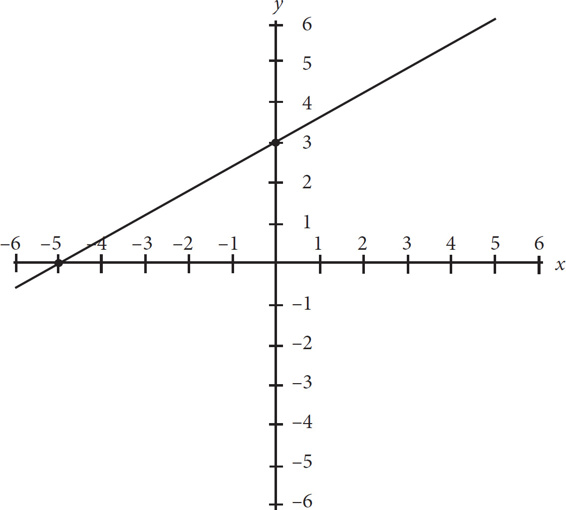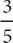# SAT Math Multiple Choice Question 108: Answer and Explanation

### Test Information

Question: 108

3.In the figure above, the graph of y = f(x) is shown. Which of the following could be the equation of f(x) ?

• A. f(x) = -x + 3
• B. f(x) = -x - 3
• C. f(x) =x - 3
• D. f(x) =x + 3